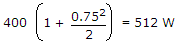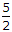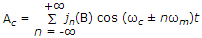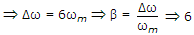# Electronics and Communication Engineering - Communication Systems

11.

A 400 W carrier is amplitude modulated with m = 0.75. The total power in AM is

 A. 400 W B. 512 W C. 588 W D. 650 W

Explanation:.

12.

c(t) and m(t) are used to generate an FM signal. If the peak frequency deviation of the generated FM signal is three times the transmission bandwidth of the AM signal, then the coefficient of the term cos [2p(1008 x 103t)] in the FM signal (in terms of the Bessel Coefficients) is

 A. 5 J4(3) B.J8(3) C.J8(4) D. 5 J4(6)

Explanation:

As, we known FM, s(t)Frequency deviation = 3 . (AM Bandwidth) = 6 fmand β= 6 is given in (d) only.

ωc + n ωm = (1008 x 103)2p2p x 106 + n. 4p x 103 = (1008 x 103).2pn = 4

So, β = 6, n = 4 hence answer is 5j4(6).

13.

Non-coherently detection is not possible for

 A. PSK B. ASK C. FSK D. both (a) and (c)

Explanation:

No answer description available for this question. Let us discuss.

14.

Tracking of extra terrestrial objects requires

 A. high transmitting power B. very sensitive receiver C. fully steerable antenna D. all of the above

Explanation:

All the three are required.

15.

Assertion (A): Free space does not interfere with normal radiation and propagation of radio waves

Reason (R): Free space has no magnetic or gravitational fields.

 A. Both A and R are correct and R is correct explanation of A B. Both A and R are correct but R is not correct explanation of A C. A is correct but R is wrong D. A is wrong but R is correct